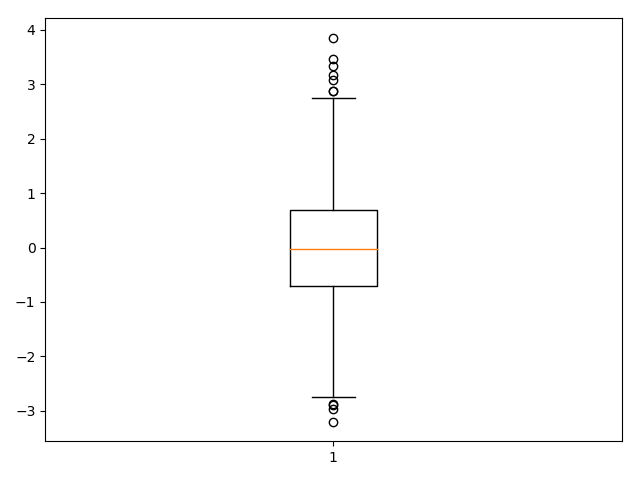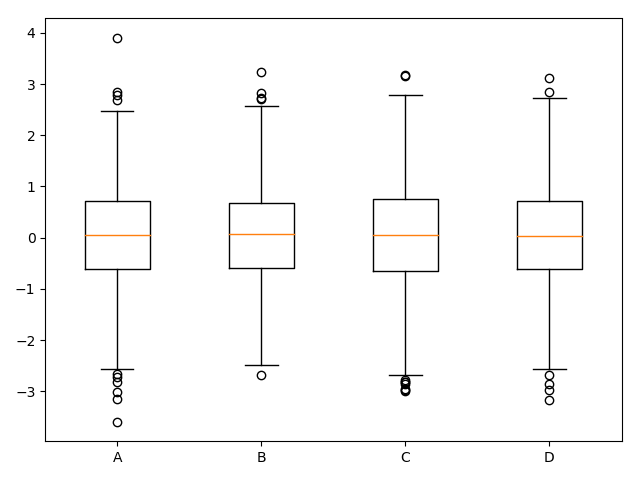# Matplotlib可视化（八）–箱形图587-王同学

,

# 概念

箱形图又称为盒须图、盒式图、箱线图

import numpy as np
import matplotlib.pyplot as plt

np.random.seed(100)
data = np.random.normal(size=1000, loc=0, scale=1)
plt.boxplot(data, sym='o', whis=1.5)
plt.show()
#sym异常值的形状
#whis代表实线长度，大就将异常值纳入一点data1 = np.random.normal(size=(1000, 4), loc=0, scale=1)
labels = list('ABCD')
plt.boxplot(data1, labels=labels)
plt.show()### 评论 抢沙发### 觉得文章有用就打赏一下文章作者

#### 支付宝扫一扫打赏#### 微信扫一扫打赏Vieu3.3主题Q Q 登 录